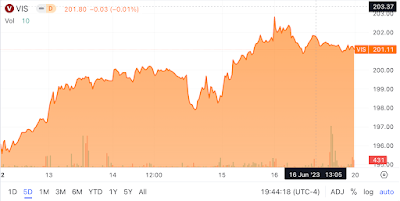Showing posts with label Linear Regression. Show all posts
Showing posts with label Linear Regression. Show all posts

## Monday, February 6, 2023

### Clorox's Poor Average Monthly Returns

Clorox makes household products such as wipes, sprays, and bleach (Exhibit 1)

Exhibit 1:Source: Clorox Website

Over the past decade, Clorox has underperformed compared to the S&P 500 Index. An analysis of the monthly returns of Clorox and the Vanguard S&P 500 Index ETF between June 2019 and January 2023 shows that Clorox continued its poor performance. Clorox's monthly return averaged 0.1%, while the Vanguard S&P 500 Index returned 1% (Exhibits 2 & 3). Even the third quartile average monthly return of 3.2% of Clorox fell below the 4.8% returned by the Vanguard S&P 500 Index ETF (Exhibits 2 & 3).

Exhibit 2: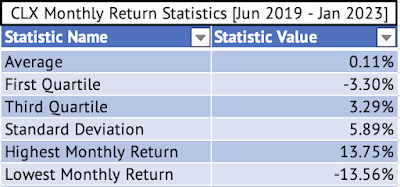Source: Data Provided by IEX Cloud, Author Calculations using Microsoft Excel

Exhibit 3: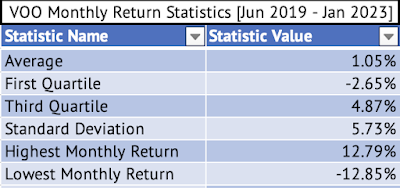Source: Data Provided by IEX Cloud, Author Calculations using Microsoft Excel
The monthly returns of Clorox and the Vanguard S&P 500 Index ETF between June 2019 and January 2023 show a low correlation of 0.24.

A rolling correlation of the monthly returns conducted using RStudio shows a low positive correlation of 0.02 between August 2020 and July 2021 (Exhibit 4)

Exhibit 4: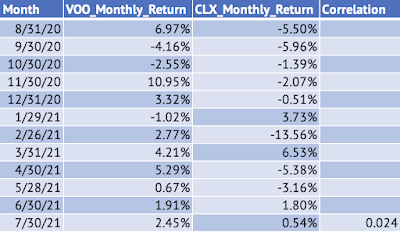Source: Data Provided by IEX Cloud, Author Calculations using Microsoft Excel, RStudio

A linear regression of the monthly returns shows a very low beta of 0.25 for Clorox. But, the p-value of 0.1 shows that the relationship may not be significant at the 95% confidence interval.

Call:

lm(formula = CLX_Monthly_Return ~ VOO_Monthly_Return, data = VOOandCLX)

Residuals:

Min        1Q    Median        3Q       Max

-0.141038 -0.033613  0.000236  0.036959  0.120780

Coefficients:

Estimate Std. Error t value Pr(>|t|)

(Intercept)        -0.001581   0.008864  -0.178    0.859

VOO_Monthly_Return  0.252497   0.153777   1.642    0.108

Residual standard error: 0.05781 on 42 degrees of freedom

Multiple R-squared:  0.06032, Adjusted R-squared:  0.03795

F-statistic: 2.696 on 1 and 42 DF,  p-value: 0.1081

Here's the graph of the monthly returns of the Vanguard S&P 500 Index ETF and Clorox (Exhibit 5) and the residuals plot from the linear regression (Exhibit 6).

Exhibit 5: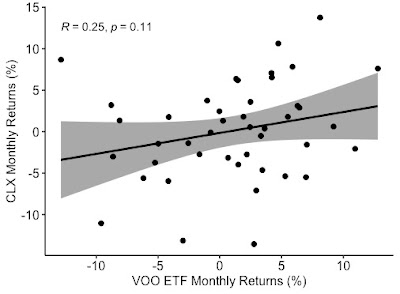Source: Data Provided by IEX Cloud, Author Calculations using Microsoft Excel, RStudio

Exhibit 6:Source: Data Provided by IEX Cloud, Author Calculations using Microsoft Excel, RStudio

## Saturday, February 4, 2023

### Change in Correlation of the Monthly Returns of Generac Holdings and the Vanguard S&P 500 Index ETF

Generac Holdings (GNRC) has a beta of 1.19 based on a linear regression model of the monthly returns of the Vanguard S&P 500 Index ETF (VOO) and Generac Holdings. The company's residential sales slowdown has pushed the stock lower over the past five months. The stock has dropped 55% compared to a 7% drop for the Vanguard S&P 500 Index ETF over the past year. This massive underperformance of the stock has led to a drop in the monthly return correlation of the Vanguard ETF and Generac Holdings.

Exhibit 1: A Generac GeneratorSource: Generac Holdings Inc.
Here's the graph of the Vanguard S&P 500 Index ETF and Generac Holdings' monthly returns (Exhibit 2).

Exhibit 2: Monthly Returns of the Vanguard S&P 500 Index ETF and Generac Holdings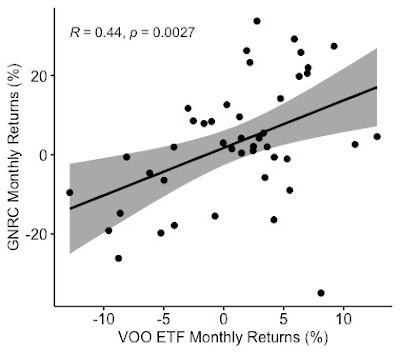Source: Data Provided by IEX Cloud, Author Calculations on Microsoft Excel, Graph Created on RStudio

The graph of the monthly returns also shows a correlation of 0.44 between the two equities.
Here are the betas of some of the stocks I have covered over the past few months (Exhibit 3)

Note: Click on each image to see an enlarged version.

Exhibit 3: Beta of Various stocks in the consumer staples, consumer discretionary, and industrial sectors.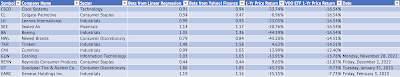Source: Data Provided by IEX Cloud, Author Calculations using Microsoft Excel and RStudio

Here's the output from the linear regression model:

Call:
lm(formula = GNRC_Monthly_Return ~ VOO_Monthly_Return, data = VOOandGNRC)

Residuals:
Min       1Q   Median       3Q      Max
-0.46400 -0.09209  0.00221  0.10001  0.28690

Coefficients:
Estimate Std. Error t value Pr(>|t|)
(Intercept)         0.01733    0.02170   0.798  0.42915
VOO_Monthly_Return  1.19915    0.37646   3.185  0.00273 **
---
Signif. codes:  0 ‘***’ 0.001 ‘**’ 0.01 ‘*’ 0.05 ‘.’ 0.1 ‘ ’ 1

Residual standard error: 0.1415 on 42 degrees of freedom
Multiple R-squared:  0.1946, Adjusted R-squared:  0.1754
F-statistic: 10.15 on 1 and 42 DF,  p-value: 0.002726

The slope of the line is the beta for the stock. In this linear regression model, the co-efficient of VOO_MonthlyReturn (1.19915) is the beta for Generac Holdings.

The monthly return statistics for Generac holdings show that the stock has a very high standard deviation of 15% in its monthly returns (Exhibit 4).

Exhibit 4: Generac Holdings Monthly Return Statistics [June 2019 - January 2023]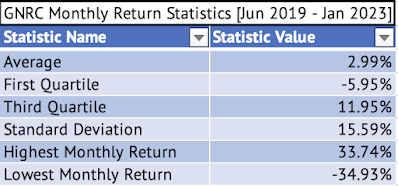Source: Data Provided by IEX Cloud, Author Calculations Using Microsoft Excel

Here are the return statistics for the Vanguard S&P 500 Index ETF during the same period (Exhibit 5).

Exhibit 5: Vanguard S&P 500 Index ETF Monthly Return Statistics  [June 2019 - January 2023]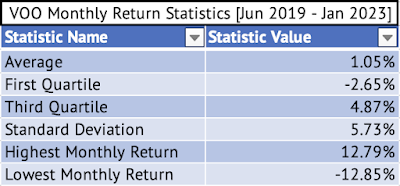Source: Data Provided by IEX Cloud, Author Calculations Using Microsoft Excel
The Vanguard S&P 500 Index ETF and Generac Holdings' monthly returns had a high positive correlation of 0.72 between October 2021 and September 2022 (Exhibit 6).

Exhibit 6: Monthly Return Correlation of the Vanguard S&P 500 Index ETF and Generac Holdings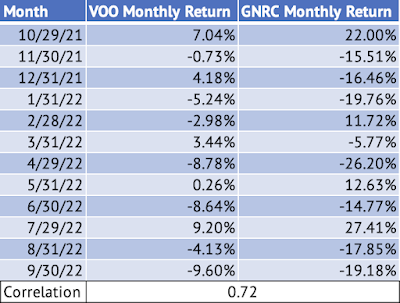Source: Data Provided by IEX Cloud, Author Calculations Using Microsoft Excel and RStudio

## Tuesday, January 31, 2023

### Why is the stock of Goodyear Tire & Rubber Co. So Volatile?

Goodyear (GT) is a very volatile stock with a beta of 1.86, measured using a linear regression model. This linear regression model used monthly returns for Goodyear and the Vanguard S&P 500 Index ETF (VOO) from June 2019 to January 2023. This beta is one of the highest I have encountered among the stocks I cover (Exhibit 1).

Image: 90% Sustainable Material Demonstration TireSource: Goodyear Tire & Rubber Co.

The stock is heavily dependent on the discretionary spending of the consumer. In a downturn, car sales drop, thus affecting Goodyear's sales. The replacement tire sales also drop in an economic slowdown, affecting the company. These might be the reasons behind the high volatility.

Note: Please click on the image to see an enlarged version.

Exhibit 1: Beta of Stocks in Industrial, Consumer Staples, Technology, and Consumer Discretionary Sectors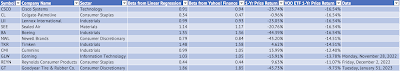Source: Data Provided by IEX Cloud, Author Calculations using Microsoft Excel & RStudio

Here's the output from the linear regression model:

Call:
lm(formula = GT_Monthly_Return ~ VOO_Monthly_Return, data = VOOandGT)

Residuals:
Min       1Q   Median       3Q      Max
-0.21099 -0.10456 -0.01782  0.07052  0.55012

Coefficients:
Estimate Std. Error t value Pr(>|t|)
(Intercept)        -0.008481   0.021853  -0.388      0.7
VOO_Monthly_Return  1.869864   0.379106   4.932 1.33e-05 ***
---
Signif. codes:  0 ‘***’ 0.001 ‘**’ 0.01 ‘*’ 0.05 ‘.’ 0.1 ‘ ’ 1

Residual standard error: 0.1425 on 42 degrees of freedom
Multiple R-squared:  0.3668, Adjusted R-squared:  0.3517
F-statistic: 24.33 on 1 and 42 DF,  p-value: 1.326e-05

The coefficient for "VOO_Monthly_Return" is the beta for the stock.  This coefficient is also the slope of the line.  The monthly returns of Goodyear have been plotted against the returns of the Vanguard S&P 500 Index ETF (Exhibit 2). Exhibit 3 shows the residuals from the linear regression mode. There is a solid positive monthly return correlation of 0.61 between the Vanguard ETF and Goodyear. There is also a significant relationship between the monthly returns of the Vanguard ETF and Goodyear, with a p-value of 1.3e-05.

Exhibit 2: Monthly Returns Plot of the Vanguard S&P 500 Index ETF and Goodyear TiresSource: Data Provided by IEX Cloud, Author Calculations Using Microsoft Excel, and Graph Plotted Using RStudio

Exhibit 3: Residuals from the Linear Regression of the Vanguard S&P 500 Index ETF and Goodyear Tires Monthly Returns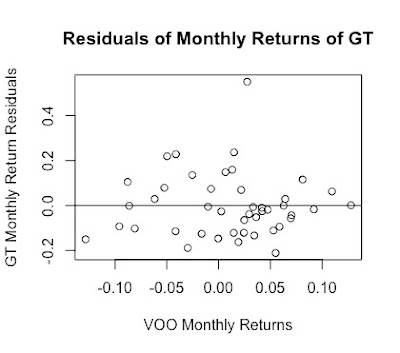Source: Data Provided by IEX Cloud, Author Calculations Using Microsoft Excel, and Graph Plotted Using RStudio

## Thursday, December 15, 2022

### J.M. Smucker's Low Correlation With The Vanguard S&P 500 Index ETF

J.M. Smucker is known for its iconic and timeless consumer staples brands (Exhibit 1)

Note: Click on each image in this blog post to view an enlarged version

Exhibit 1: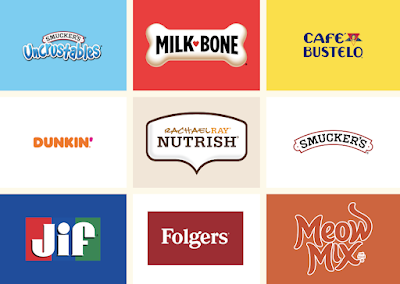Brands Owned by J.M. Smucker & Co. (Source: J.M. Smucker)

Here's the histogram of monthly returns for J.M. Smucker between June 2019 and November 2022 (Exhibit 2).

Exhibit 2: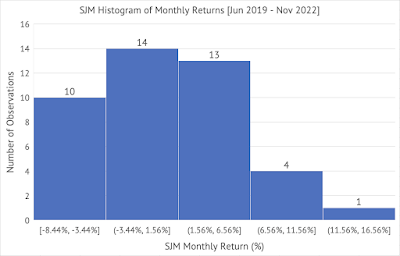J.M. Smucker (SJM) Histogram of Monthly Returns (Source: Data provided by IEX Cloud, author calculations & graph using Microsoft Excel)

The average monthly return for J.M. Smucker is less than the Vanguard S&P 500 Index ETF (Exhibit 3).
Exhibit 3: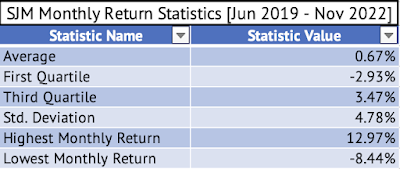J.M. Smucker Monthly Return Statistics - Average, First Quartile, Third Quartile, Standard Deviation, Highest Monthly Return, Lowest Monthly Return (Source: Data provided by IEX Cloud, author calculations & graph using Microsoft Excel)

J.M. Smucker had a lower standard deviation than the Vanguard ETF during this period (Exhibit 4). A company with a lower standard deviation than the well-diversified ETF, a measure of volatility, is an infrequent occurrence.

Exhibit 4: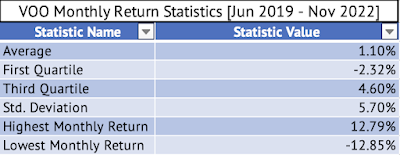Vanguard S&P 500 Index ETF Monthly Return Statistics - Average, First Quartile, Third Quartile, Standard Deviation, Highest Monthly Return, Lowest Monthly Return (Source: Data provided by IEX Cloud, author calculations & graph using Microsoft Excel)

Here's a graph of the monthly returns of the Vanguard ETF (x-axis) and J.M.Smucker (y-axis) with the fitted regression line (Exhibit 5).
Exhibit 5: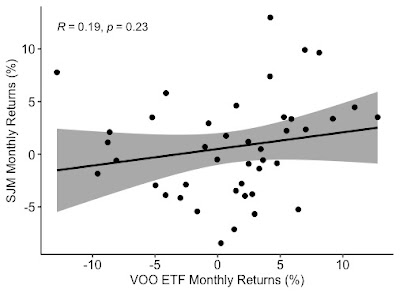Monthly Return Graph of the Vanguard S&P 500 Index ETF and J.M. Smucker (Source: Data provided by IEX Cloud, author calculations & graph using Microsoft Excel & RStudio)

The correlation of the monthly returns between June 2019 and November 2022 between the Vanguard ETF and J.M. Smucker is a low 0.19 (Exhibit 5). The fitted linear regression line has a p-value of 0.23, indicating that the relationship is insignificant at the 95% confidence interval.

The fitted linear regression line has a p-value of 0.23, indicating that the relationship is insignificant at the 95% confidence interval. Here's the output from the linear regression:

> summary(lmVOOSJM)

Call:
lm(formula = SJM_Monthly_Return ~ VOO_Monthly_Return, data = VOOandSJM)

Residuals:
Min        1Q    Median        3Q       Max
-0.089842 -0.034389  0.002403  0.022171  0.118077

Coefficients:
Estimate  Std. Error t value  Pr(>|t|)
(Intercept)        0.005011   0.007476   0.670    0.507
VOO_Monthly_Return 0.157911   0.130216   1.213    0.232

Residual standard error: 0.04755 on 40 degrees of freedom
Multiple R-squared:  0.03546, Adjusted R-squared:  0.01135
F-statistic: 1.471 on 1 and 40 DF,  p-value: 0.2324

The beta for J.M. Smucker is 0.15, but the high p-value is a concern. This beta (the coefficient of VOO_Monthly_Return) may not be the true value. Yahoo Finance has calculated a beta of 0.24.

## Sunday, December 4, 2022

### Mothly Return Volatility (Beta) of Reynolds Consumer Products

Reynolds Consumer Products (REYN) makes many iconic household products, such as Reynolds Wrap, Hefty waste bags, and FreshLock zipper bags [Exhibit 1]

Exhibit 1: Some of the Products Made by Reynolds Consumer Products Co.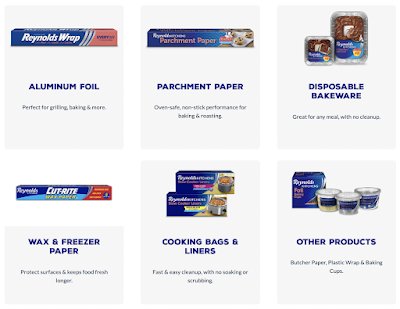Reynolds Consumer Products Source: Reynolds brands

I analyzed the monthly return of Reynolds (REYN) between February 2020 and November 2022. Here's the histogram of the monthly returns (click on the image to see an enlarged version) [Exhibit 2]:

Exhibit 2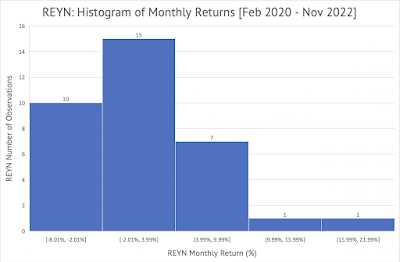Source: Data Provided by IEX Cloud, Author Calculations and Graphs Using Microsoft Excel

Here's the graph of the monthly returns of the Vanguard S&P 500 Index ETF (VOO) on the x-axis and Reynold's monthly returns on the y-axis [Exhibit 3]:

Exhibit 3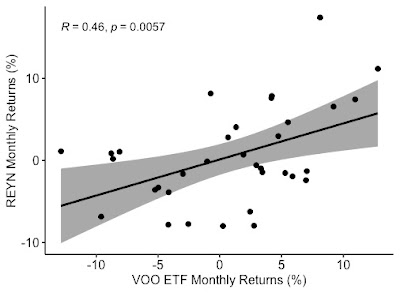Source: Data Provided by IEX Cloud, Graph Created using RStudio

The Pearson correlation of the monthly returns is a positive 0.46. This correlation value can be considered to have medium strength. This correlation is statistically significant at the 95% confidence interval with a p-value of 0.0057.

A linear regression of the monthly returns of Reynolds and the Vanguard S&P 500 Index ETF yields a beta value of 0.44. This beta value means that for every 1% change in the value of the Vanguard ETF, on average, Reynolds' stock will change by 0.44%. Yahoo Finance also shows a beta of 0.44 [Exhibit 4]

Exhibit 4

Source: Yahoo Finance

The adjusted R-squared value provided by the linear regression is 0.19. This adjusted R-squared value indicates that about 19% of Reynold's monthly returns are explained by the monthly returns of the Vanguard S&P 500 Index ETF.

Here's the output from the linear regression model constructed using RStudio:

Call:

lm(formula = REYN_Monthly_Return ~ VOO_Monthly_Return, data = VOOandREYN_MonthlyReturns)

Residuals:

Min        1Q    Median        3Q       Max

-0.092919 -0.037524 -0.003499  0.037494  0.137349

Coefficients:

Estimate Std. Error t value Pr(>|t|)

(Intercept)        0.001072   0.009234   0.116  0.90828

VOO_Monthly_Return 0.440311   0.148653   2.962  0.00572 **

---

Signif. codes:  0 ‘***’ 0.001 ‘**’ 0.01 ‘*’ 0.05 ‘.’ 0.1 ‘ ’ 1

Residual standard error: 0.05329 on 32 degrees of freedom

Multiple R-squared:  0.2152, Adjusted R-squared:  0.1907

F-statistic: 8.773 on 1 and 32 DF,  p-value: 0.005722

The p-value is significant at a 95% confidence interval with a value of 0.005722.

Here's the residuals plot for the linear regression between Reynolds Consumer Products and the Vanguard S&P 500 Index ETF [Exhibit 5]:

Exhibit 5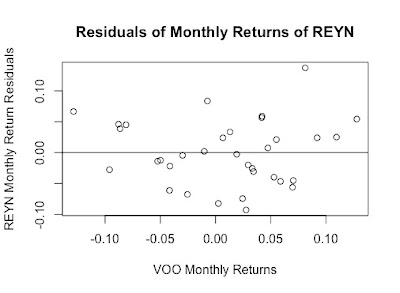Residuals Plot for Linear Regression of the monthly returns of the Vanguard S&P 500 Index as the independent variable and Reynold Consumer Products (Source: Data Provided by IEX Cloud, Graph Created using RStudio)

Here are the average, first quartile, third quartile, and standard deviation of Reynold's monthly returns [Exhibit 6]:

Exhibit 6

Here are the average, first quartile, third quartile, and standard deviation of the Vanguard S&P 500 Index ETF [Exhibit 7]:

## Monday, November 28, 2022

### Corning's Volatility Compared to the Vanguard S&P 500 Index ETF

Here's the histogram of Corning's (GLW) monthly returns between June 2019 and October 2022 (Click on the image to see a larger version):

Exhibit: Corning's Monthly Returns Fell at or below 2.56% for the Majority of the Months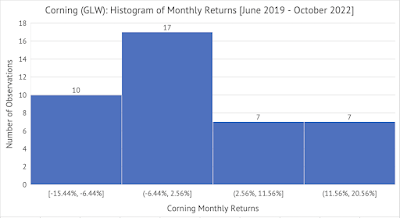Data Provided by IEX Cloud, Author Calculations

There were 14 months between June 2019 and October 2022 when Corning's monthly returns were greater than 2.56%.  There were seven months when the monthly returns were greater than or equal to 11.5%.

Corning's monthly returns have a high positive correlation of 0.66 with the monthly returns of the Vanguard S&P 500 Index ETF (VOO).

Exhibit: The Vanguard S&P 500 Index and Corning Monthly Returns [June 2019 - October 2022]Data Provided by IEX Cloud, Monthly Returns Calculated by the Author, Graph using RStudio

A linear regression of the Vanguard S&P 500 Index ETF and Corning's monthly returns yields a beta of 1.03 for Corning. Corning's average monthly return will mirror the Vanguard S&P 500 Index ETF. Corning may not help diversify a portfolio and will not protect against the market's volatility.

Here's the linear regression model:

> # Conduct the Linear Regression of the Monthly Returns Between \$VOO and \$GLW

> lmVOOGLW = lm(GLW_Monthly_Return~VOO_Monthly_Return, data = VOOandGLW)

> # Present the summary of the results from the linear regression

> summary(lmVOOGLW)

Call:

lm(formula = GLW_Monthly_Return ~ VOO_Monthly_Return, data = VOOandGLW)

Residuals:

Min        1Q      Median     3Q       Max

-0.118363 -0.050928 -0.009998  0.041106  0.187435

Coefficients:

Estimate     Std. Error   t value   Pr(>|t|)

(Intercept)        -0.003778     0.010818    -0.349    0.729

VOO_Monthly_Return  1.039148     0.188256     5.520    2.4e-06 ***

---

Signif. codes:  0 ‘***’ 0.001 ‘**’ 0.01 ‘*’ 0.05 ‘.’ 0.1 ‘ ’ 1

Residual standard error: 0.06823 on 39 degrees of freedom

Multiple R-squared:  0.4386, Adjusted R-squared:  0.4242

F-statistic: 30.47 on 1 and 39 DF,  p-value: 2.403e-06

The coefficient for VOO_Monthly_Return [1.039148] is the beta for Corning.  The p-value is significant at a 95% confidence interval. The adjusted R-squared is 0.42, which means about 42% of Corning's average monthly return is explained by the Vanguard S&P 500 Index ETF returns.

## Saturday, November 26, 2022

### Monthly Return Comparison Between Cummins and Vanguard S&P 500 Index ETF

The following chart shows the Vanguard S&P 500 Index ETF (VOO) monthly returns on the x-axis and Cummins (CMI) on the y-axis. The regression line on the graph shows a steep slope, and the Pearson correlation value is 0.7. This value shows a very strong correlation between the monthly returns of the Vanguard S&P 500 Index ETF and Cummins. The p-value is significant at a 95% confidence interval. Cummins has returned 13.9% in the past year, while the Vanguard S&P 500 Index ETF has returned a -12.4%.

Exhibit: Monthly Returns of Vanguard S&P 500 Index ETF and Cummins [June 2019 - October 2022]

> # Create a new Graph of \$VOO and \$CMI Monthly Returns as Percentages
> ggscatter(df1, x = 'VOO_Monthly_Return', y = 'CMI_Monthly_Return',
+           add = "reg.line", conf.int = TRUE,
+           cor.coef = TRUE, cor.method = "pearson",
+           xlab = "VOO ETF Monthly Returns (%)", ylab = "CMI Monthly +           Returns (%)")

A linear regression of the monthly returns of the Vanguard S&P 500 Index ETF and Cummins shows the beta (coefficient of Vanguard S&P 500 Index ETF) for Cummins' monthly returns compared to the Vanguard ETF.

# Conduct the Linear Regression of the Monthly Returns Between \$VOO and \$CMI
lmVOOCMI = lm(CMI_Monthly_Return~VOO_Monthly_Return, data = VOOandCMI)
# Present the summary of the results from the linear regression
summary(lmVOOCMI)

Call:
lm(formula = CMI_Monthly_Return ~ VOO_Monthly_Return, data = VOOandCMI)

Residuals:
Min        1Q    Median        3Q       Max
-0.123268 -0.051037  0.004537  0.047062  0.115727

Coefficients:
Estimate Std. Error t value Pr(>|t|)
(Intercept)        0.005104   0.009226   0.553    0.583
VOO_Monthly_Return 0.993327   0.160541   6.187 2.84e-07 ***
---
Signif. codes:  0 ‘***’ 0.001 ‘**’ 0.01 ‘*’ 0.05 ‘.’ 0.1 ‘ ’ 1

Residual standard error: 0.05819 on 39 degrees of freedom
Multiple R-squared:  0.4954, Adjusted R-squared:  0.4824
F-statistic: 38.28 on 1 and 39 DF,  p-value: 2.845e-07

Cummins has a beta of 0.99 (nearly 1). Given this beta, Cummins, on average, moves in line with the S&P 500 Index. The adjusted R-squared value of 0.48 shows that about 48% of Cummins' monthly returns can be attributed to the returns of the S&P 500 Index. The p-value of 2.84e-07 shows that the regression analysis results are significant at the 95% confidence interval.

The average monthly return for Cummins between June 2019 and October 2022 was 1.49%. A one-sample t-test shows that the monthly return falls between -1.05% and 4.04%. But, the p-value of 0.99 is much higher than 0.05. This t-test may not be significant in the 95% confidence interval.

#
# One Sample t-test of \$CMI average monthly returns.
# Step 1:
# Copy Dataframe Column CMI_Monthly_Return into a List
#
CMI_Monthly_Return_Col <- c(VOOandCMI['CMI_Monthly_Return'])
# CMI_Monthly_Return_Col is a list object, but needs to be numeric
# for qqnorm to work.
#
typeof(CMI_Monthly_Return_Col)
# Convert List Object into a Column of doubles as as.numeric and unlist
#
y_CMI_Monthly_Return_Col <- as.numeric(unlist(CMI_Monthly_Return_Col))
typeof(y_CMI_Monthly_Return_Col)
# Let’s check if the data comes from a normal distribution
# using a normal quantile-quantile plot.
# Source: https://cran.r-project.org/web/packages/distributions3/vignettes/one-sample-t-test.html

Exhibit: Check if Cummins' Monthly Returns are Normally Distributed before doing a t-test

#
qqnorm(y_CMI_Monthly_Return_Col)
qqline(y_CMI_Monthly_Return_Col)
# Conduct a t-test to see if the population mean is 1.49% [0.0149]
#
t.test(y_CMI_Monthly_Return_Col, mu = .0149)

One Sample t-test

data:  y_CMI_Monthly_Return_Col
t = 0.0045069, df = 40, p-value = 0.9964
alternative hypothesis: true mean is not equal to 0.0149
95 percent confidence interval:
-0.01057188  0.04048574
sample estimates:
mean of x
0.01495693

## Tuesday, September 27, 2022

### Boeing's Monthly Return Volatility Compared to the Vanguard S&P 500 Index ETF From June 2019 to August 2022

Given its dominant position in the aerospace market, one would think Boeing's (BA) monthly returns would be less volatile than the S&P 500 index (VOO). But, Boeing has endured a lot in the past few years. First came the trade war with China that froze Boeing out of the second-largest aerospace market in the world.  Then came the COVID-19 pandemic that grounded airlines worldwide and brought Boeing to its knees. We did not even talk about the 737 Max plane crash in Ethiopia that kicked off the disastrous few years for Boeing.

Boeing has never fully recovered from either the trade war or the pandemic. Boeing remains frozen out of the Chinese market, and airlines are only now seeing air travel return close to pre-pandemic levels (Exhibit 1).

Exhibit 1: TSA Checkpoint Travel Number September 17, 2022 - September 26, 2022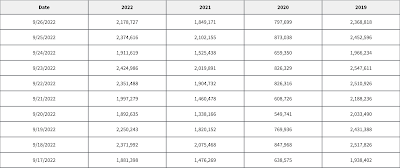TSA Checkpoint Travel Numbers (Source: TSA.GOV)

Now, the world is grappling with slowing growth due to high inflation and interest rates, which is putting further pressure on Boeing. By the looks of it, Boeing stock may take a decade or more to recover its losses if it ever recovers. Boeing's stock has dropped from \$440 in March 2019 to \$127 as of September 27 - a loss of 71%.
Due to these massive crises, Boeing's stock returns have become unhinged from that of the S&P 500 index. A linear regression of the monthly returns of the Vanguard S&P 500 Index and Boeing yields a very high beta of 1.35 (slope of the regression line). The value of 1.35 is the coefficient of the monthly returns of the Vanguard S&P 500 Index ETF (VOO).  Yahoo Finance displays a beta of 1.36 based on 5-year monthly returns.  One can expect any change in the Vanguard ETF to be magnified by Boeing.  For every 1% change in monthly returns of the S&P 500 index, Boeing's monthly returns are expected to change by 1.35%. Also, just 25% (Adjusted R-Squared in the RStudio output below) of Boeing's returns are explained by the monthly returns of the S&P 500 Index.

Exhibit: Vanguard S&P 500 Index ETF and Boeing Monthly Returns [June 2019 - August 2022]Vanguard S&P 500 Index ETF and Boeing Monthly Returns [June 2019 - August 2022] (Source: Data Provided by IEX Cloud, Author Calculations Using RStudio)

Here's the output from the linear regression conducted on RStudio:

> lmBAVOO = lm(BA_Monthly_Return~VOO_Monthly_Return, data = VOOandBA)

> summary(lmBAVOO)

Call:

lm(formula = BA_Monthly_Return ~ VOO_Monthly_Return, data = VOOandBA)

Residuals:

Min       1Q   Median       3Q      Max

-0.26036 -0.07433 -0.00562  0.07323  0.33452

Coefficients:

Estimate Std. Error t value Pr(>|t|)

(Intercept)        -0.02348    0.02007  -1.169 0.249682

VOO_Monthly_Return  1.35442    0.36247   3.737 0.000628 ***

---

Signif. codes:  0 ‘***’ 0.001 ‘**’ 0.01 ‘*’ 0.05 ‘.’ 0.1 ‘ ’ 1

Residual standard error: 0.1229 on 37 degrees of freedom

Multiple R-squared:  0.274, Adjusted R-squared:  0.2543

F-statistic: 13.96 on 1 and 37 DF,  p-value: 0.0006279

## Monday, September 5, 2022

### Linear Regression of Monthly Returns of Colgate-Palmolive against Vanguard S&P 500 Index ETF

Here is the graph of monthly returns of Colgate-Palmolive (CL) plotted against Vanguard S&P 500 Index ETF (VOO):

Exhibit 1: Monthly Returns of Colgate-Palmolive and Vanguard S&P 500 Index ETF [June 2019 -  August 2022]

(Source: RStudio, ggplot, Data Provided by IEX Cloud)

Results of the linear regression of monthly returns of Colgate-Palmolive against Vanguard S&P 500 Index ETF:

> VOOandCL <- read_excel("/CL_VOO_LM_September_2022.xlsx", sheet = "Sheet1")
lmCLVOO = lm(CL_Monthly_Return~VOO_Monthly_Return, data = VOOandCL)

> summary(lmCLVOO)

Call:
lm(formula = CL_Monthly_Return ~ VOO_Monthly_Return, data = VOOandCL)

Residuals:
Min        1Q    Median        3Q       Max
-0.084505 -0.025451  0.002263  0.028820  0.122836

Coefficients:
Estimate Std. Error t value Pr(>|t|)
(Intercept)        0.0002236  0.0067940   0.033  0.97392
VOO_Monthly_Return 0.3471172  0.1226847   2.829  0.00749 **
---
Signif. codes:  0 ‘***’ 0.001 ‘**’ 0.01 ‘*’ 0.05 ‘.’ 0.1 ‘ ’ 1

Residual standard error: 0.04161 on 37 degrees of freedom
Multiple R-squared:  0.1779, Adjusted R-squared:  0.1557
F-statistic: 8.005 on 1 and 37 DF,  p-value: 0.007492

The slope of the regression corresponds to the beta of the stock. In this case, Colgate-Palmolive has a beta of 0.34.
The adjusted R-squared is 0.15. About 15% of Colgate-Palmolive's return is explained by the returns of the S&P 500 index.
Colgate-Palmolive can protect a portfolio against market volatility since it has a beta value substantially less than 1.

## Saturday, September 3, 2022

### Linear Regression of Monthly Returns of Lennox International against Vanguard S&P 500 Index ETF

Here is the graph of monthly returns of Lennox International (LII) plotted against Vanguard S&P 500 Index ETF (VOO):

Exhibit 1: Monthly Returns of Lennox International and Vanguard S&P 500 Index ETF [June 2019 -  August 2022

(Source: RStudio, ggplot, Data Provided by IEX Cloud)

Results of the linear regression of monthly returns of Lennox International against Vanguard S&P 500 Index ETF:

VOOandLII <- read_excel("/LII_VOO_LM_September_2022.xlsx", sheet = "Sheet1")

lmLIIVOO = lm(LII_Monthly_Return~VOO_Monthly_Return, data = VOOandLII)

> summary(lmLIIVOO)

Call:
lm(formula = LII_Monthly_Return ~ VOO_Monthly_Return, data = VOOandLII)

Residuals:
Min        1Q    Median        3Q       Max
-0.090246 -0.052733 -0.001907  0.040310  0.108446

Coefficients:
Estimate Std. Error t value Pr(>|t|)
(Intercept)        -0.010082   0.009416  -1.071    0.291
VOO_Monthly_Return  0.995050   0.170040   5.852 9.97e-07 ***
---
Signif. codes:  0 ‘***’ 0.001 ‘**’ 0.01 ‘*’ 0.05 ‘.’ 0.1 ‘ ’ 1

Residual standard error: 0.05767 on 37 degrees of freedom
Multiple R-squared:  0.4807, Adjusted R-squared:  0.4666
F-statistic: 34.24 on 1 and 37 DF,  p-value: 9.966e-07

The slope of the regression corresponds to the beta of the stock. In this case, Lennox International has a beta of 0.995. Lennox's beta is close to the S&P 500 beta, so Lennox will move in line with the market.

The adjusted R-squared is 0.46. About 46% of Lennox's return is explained by the returns of the S&P 500 index.

### The Industrials Sector is on a Tear

The Vanguard Industrials Index ETF ( VIS ) touched a 52-week high of \$202.86 on Friday, June 16 (Exhibit 1) .   Exhibit 1: Vanguard Industr...